# Perform three types of t-test in Python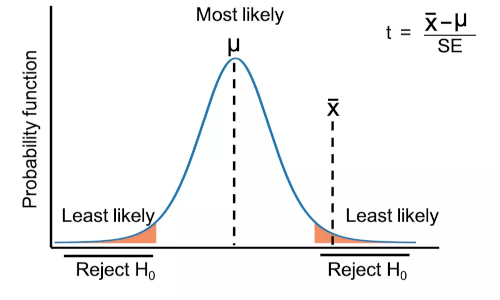## Student’s t-test

• Student’s t-test or t-test is a parametric statistical method used for comparing the means between two different groups (two-sample) or with the specific value (one-sample).
• In t-test, test statistic follows the t-distribution (type of continuous probability distribution) under the null hypothesis.
• t-distribution is first proposed by William Sealy Gosset and published under the fictitious name of “Student” in Biometrika scientific journal. Hence, t-distribution is also known as Student’s t-distribution.
• In contrast to the z-test, which requires a larger sample size, the t-test is specially developed for the small sample size data (n ≤ 30). t-test is also applies for extremely small sample size data (n ≤ 5).
• t-test has three main types: One Sample t-test, two sample t-test (unpaired or independent), and paired t-test.

## Types of t-test

### One Sample t-test

• One Sample t-test (single sample t-test) is used to compare the sample mean (a random sample from a population) with the specific value (hypothesized or known mean of the population).
• For example, a ball has a diameter of 5 cm and we want to check whether the average diameter of the ball from the random sample (e.g. 50 balls) picked from the production line differs from the known size.

#### Assumptions

• Dependent variable should have an approximately normal distribution (Shapiro-Wilks Test)
• Observations are independent of each other

Note: One sample t-test is relatively robust to the assumption of normality when the sample size is large (n ≥ 30)

#### Hypotheses

• Null hypothesis: Sample mean is equal to the hypothesized or known population mean
• Alternative hypothesis: Sample mean is not equal to the hypothesized or known population mean (two-tailed or two-sided)
• Alternative hypothesis: Sample mean is either greater or lesser to the hypothesized or known population mean (one-tailed or one-sided)

#### Formula

One Sample t-test formula,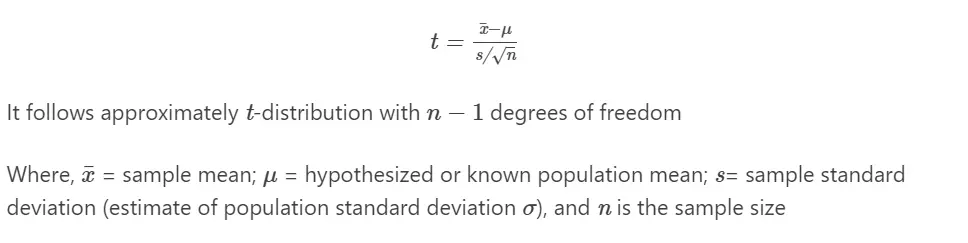#### Calculate one sample t-test in Python

Note: If you have your own dataset, you should import it as pandas dataframe. Learn how to import data using pandas

Perform one sample t-test using SciPy,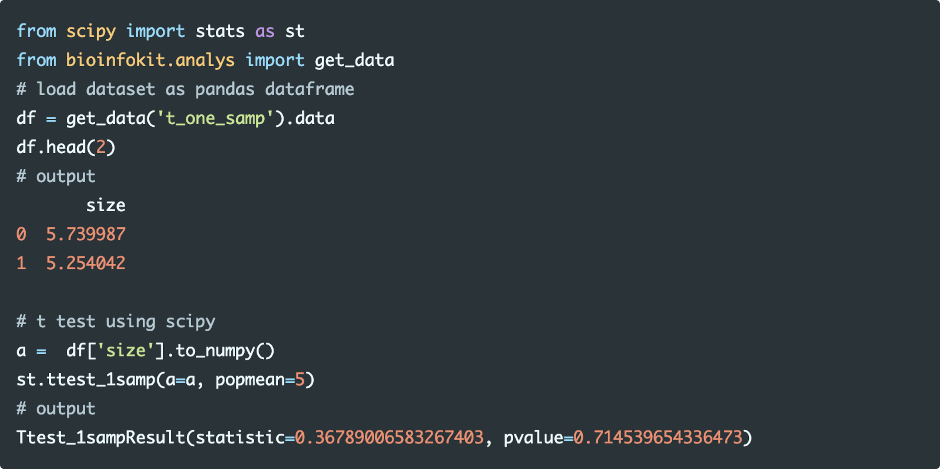Run the code in colab

Perform one sample t-test using bioinfokit,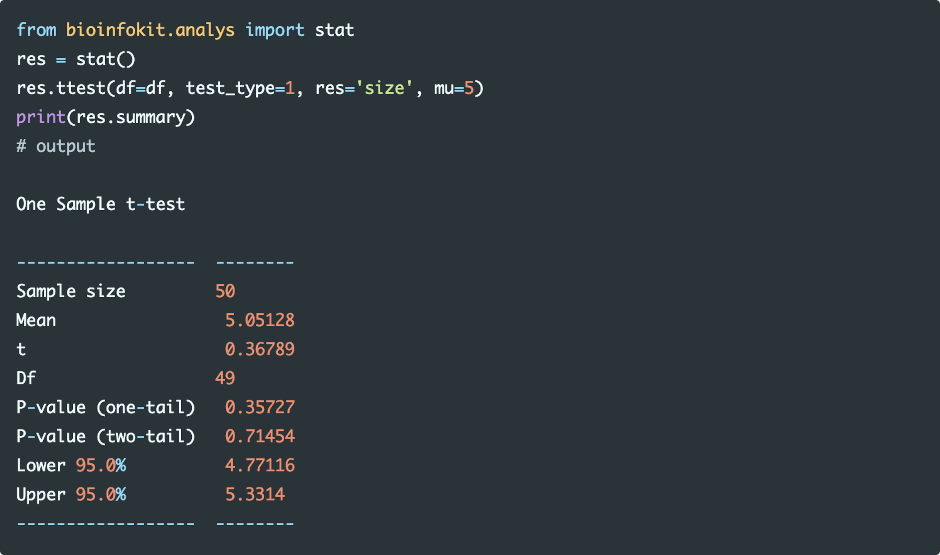Run the code in colab

#### Interpretation

The p value obtained from the one sample t-test is not significant (p > 0.05), and therefore, we conclude that the average diameter of the balls in a random sample is equal to 5 cm.

### Two sample t-test (unpaired or independent t-test)

• The two-sample (unpaired or independent) t-test compares the means of two independent groups, determining whether they are equal or significantly different.
• In two sample t-test, usually, we compute the sample means from two groups and derives the conclusion for the population’s means (unknown means) from which two groups are drawn.
• For example, we have two different plant genotypes (genotype A and genotype B) and would like to compare if the yield of genotype A is significantly different from genotype B

#### Hypotheses

• Null hypothesis: Two group means are equal
• Alternative hypothesis: Two group means are different (two-tailed or two-sided)
• Alternative hypothesis: Mean of one group either greater or lesser than another group (one-tailed or one-sided)

#### Assumptions

• Observations in two groups have an approximately normal distribution (Shapiro-Wilks Test)
• Homogeneity of variances (variances are equal between treatment groups) (Levene or Bartlett Test)
• The two groups are sampled independently from each other from the same population

Note: Two sample t-test is relatively robust to the assumption of normality and homogeneity of variances when sample size is large (n ≥ 30) and there are equal number of samples (n1 = n2) in both groups.

If the sample size small and does not follow the normal distribution, you should use non-parametric Mann-Whitney U test (Wilcoxon rank sum test)

#### Formula

Two sample (independent) t-test formula,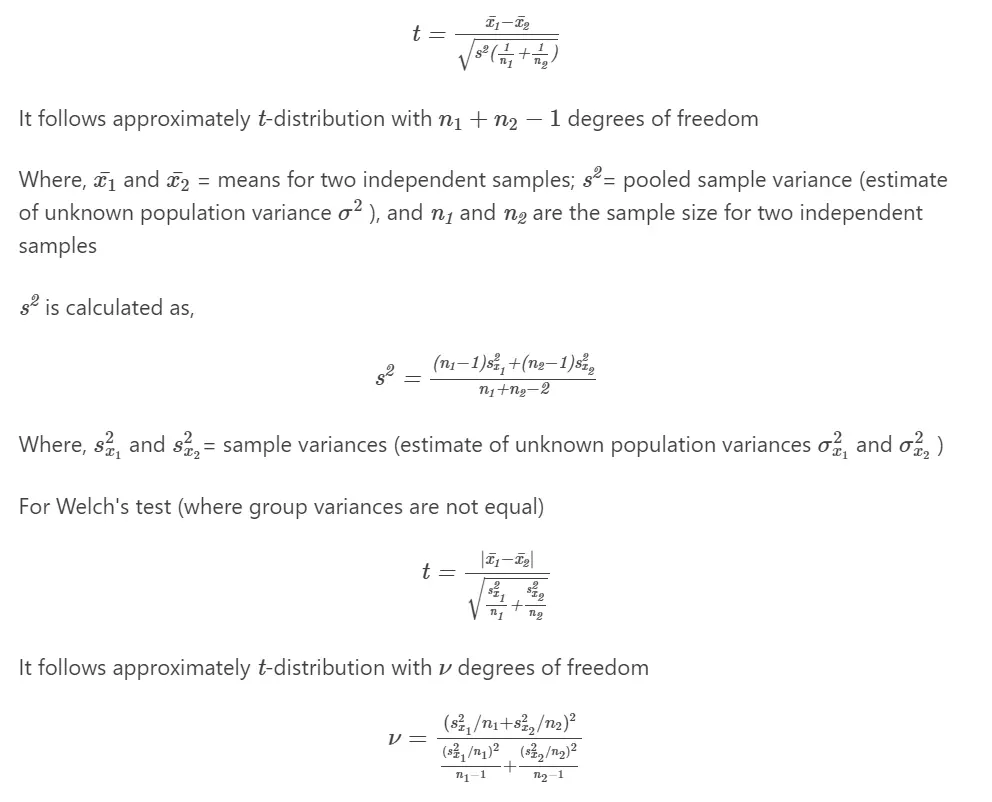If the variances are equal, the two sample t-test and Welch’s test (unequal variance t-test) perform equally (in terms of type I error rate) and have similar power.

#### Calculate Two sample t-test in Python

Perform two sample t-test using SciPy,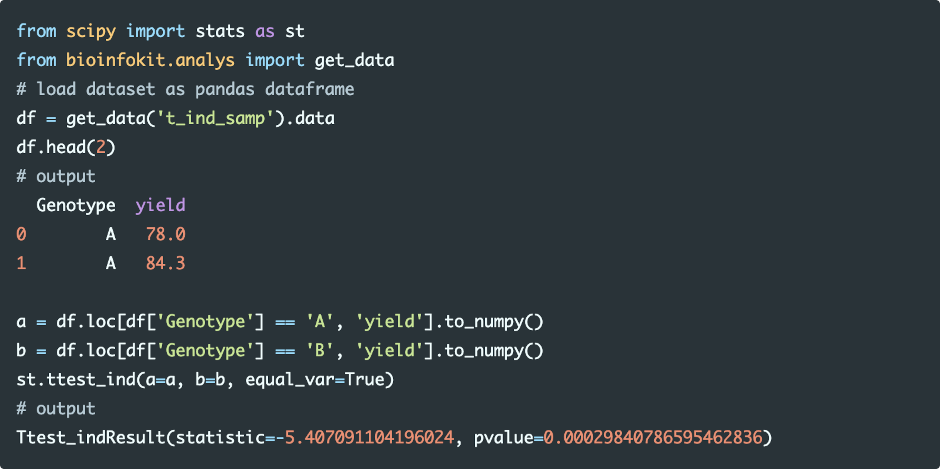Run the code in colab

Perform two sample t-test using bioinfokit,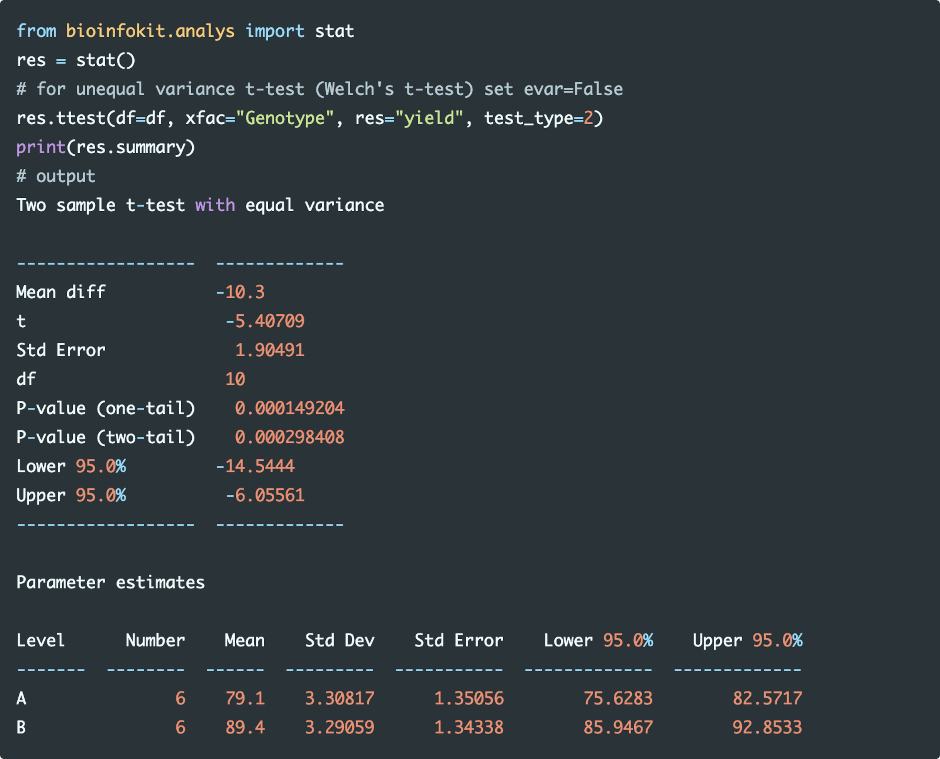Run the code in colab

Note: Even though you can perform a t-test when the sample size is unequal between two groups, it is more efficient to have an equal sample size in two groups to increase the power of the t-test.

#### Interpretation

The p value obtained from the t-test is significant (p < 0.05), and therefore, we conclude that the yield of genotype A is significantly different than genotype B.

### Paired t-test (dependent t-test)

• Paired t-test used to compare the differences between the pair of dependent variables for the same subject
• For example, we have plant variety A and would like to compare the yield of A before and after the application of some fertilizer
• Note: Paired t-test is a one sample t-test on the differences between the two dependent variables

#### Hypotheses

• Null hypothesis: There is no difference between the two dependent variables (difference=0)
• Alternative hypothesis: There is a difference between the two dependent variables (two-tailed or two-sided)
• Alternative hypothesis: Difference between two response variables either greater or lesser than zero (one-tailed or one-sided)

#### Assumptions

• Differences between the two dependent variables follows an approximately normal distribution (Shapiro-Wilks Test)
• Independent variable should have a pair of dependent variables
• Differences between the two dependent variables should not have outliers
• Observations are sampled independently from each other

#### Formula

Paired t-test formula,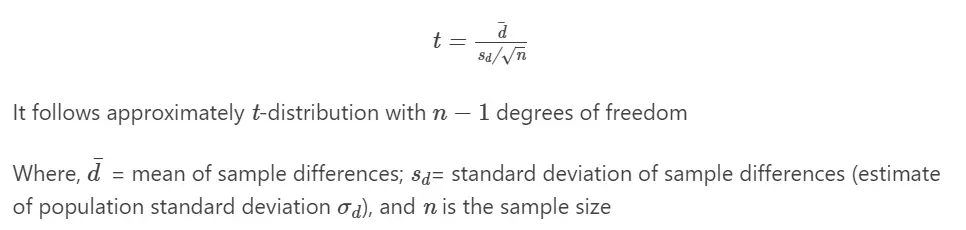Perform Paired t-test,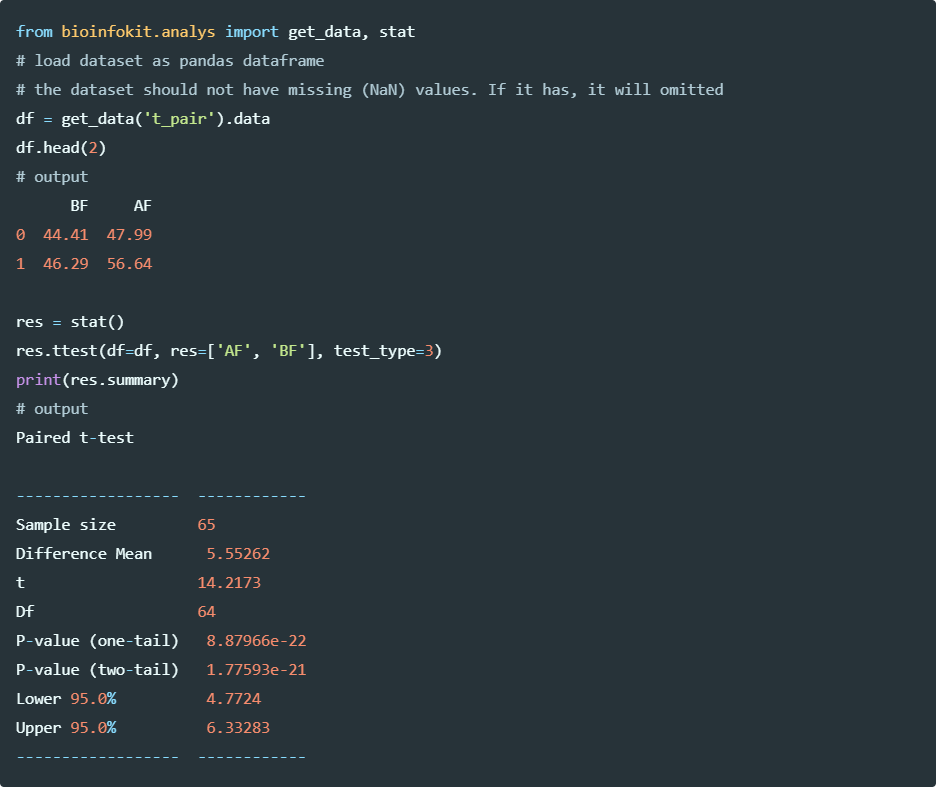Run the code in colab

#### Interpretation

The p value obtained from the t-test is significant (p < 0.05), and therefore, we conclude that the yield of plant variety A significantly increased by the application of fertilizer.

Note: If you have partially paired data, you can use an independent t-test by treating two dependent variables as two different samples or drop all unpaired observations for performing paired t-test. But, both ad hoc approaches are not appropriate as it does not follow the basic requirement and may lead to biased estimate of the variance and loss of information 6.

## Sample size recommendations for t-test

• The t-test can be applied for the extremely small sample size (n = 2 to 5) provided the effect size is large and data follows the t-test assumptions. Remember, a larger sample size is preferred over small sample sizes.
• For paired t-test, it is advisable to have a high within-pair correlation (r > 0.8) to get a high statistical power (>80%) for small sample size data.
• t-test is relatively robust to the assumption of normality and homogeneity of variances when the sample size is large (n ≥ 30).
1. Virtanen P, Gommers R, Oliphant TE, Haberland M, Reddy T, Cournapeau D, Burovski E, Peterson P, Weckesser W, Bright J, van der Walt SJ. SciPy 1.0: fundamental algorithms for scientific computing in Python. Nature methods. 2020 Mar;17(3):261-72.
2. Kim TK, Park JH. More about the basic assumptions of t-test: normality and sample size. Korean journal of anesthesiology. 2019 Aug;72(4):331.
3. Schober P, Vetter TR. Two-sample unpaired t tests in medical research. Anesthesia & Analgesia. 2019 Oct 1;129(4):911.
4. Zabell SL. On student’s 1908 article “the probable error of a mean”. Journal of the American Statistical Association. 2008 Mar 1;103(481):1-7.
5. De Winter JC. Using the Student’s t-test with extremely small sample sizes. Practical Assessment, Research, and Evaluation. 2013;18(1):10.
6. Guo B, Yuan Y. A comparative review of methods for comparing means using partially paired data. Statistical methods in medical research. 2017 Jun;26(3):1323-40.
7. Ruxton GD. The unequal variance t-test is an underused alternative to Student’s t-test and the Mann–Whitney U test. Behavioral Ecology. 2006 Jul 1;17(4):688-90.